Email : info@epmstrategy.com | Call Us Now : 479-321-3977
:: Home :: Sitemap ::

# Power BI Round Function in DAX

BY: David Rohlfs

Introduction

Data will always start very messy, and that is one of the exciting things that every report builder gets to navigate. Even when data is clean, there are usually some ways that a report builder can make data more presentable, and one of these ways is using the Round Function in DAX.

In this blog we will go over when to use the round function and a walkthrough of creating the DAX formula for this function.

When to use it

The Round function is different than many other functions in DAX because it is changing the data that you give it. Because of this it is advised that you don’t use the round function unless you specify this in your graph or report. For instance, in this graph I used the round function, so I changed the title to make it clear that the X-Axis was rounded.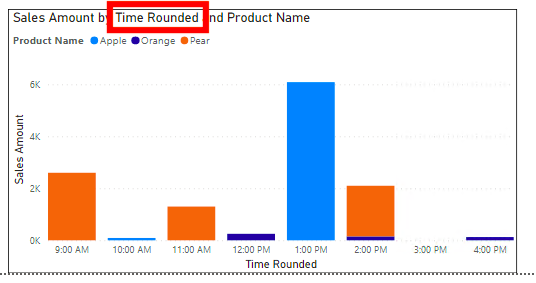The round function is very helpful when you have a wide range of data and only want to see trends in data and not specific data points. Because of this, I have mostly used this function when talking about time and wanted to show a general idea of what the data was depicting.

This is an example of a graph before it has the round function applied to it: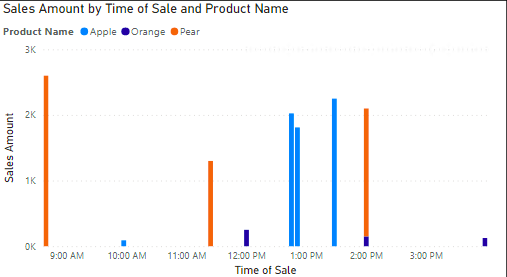And this is an example of the same graph after a round function has been applied: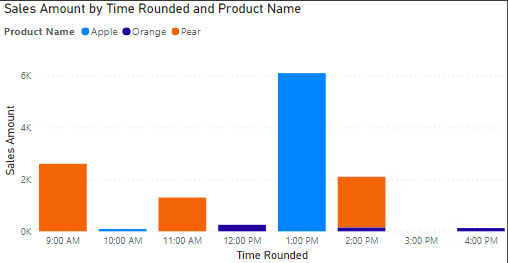This is what the original field and the rounded field look like next to each other.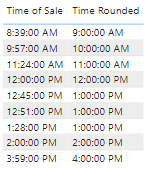You can see that I am not getting the most accurate data or keeping the data as untouched as possible, but what I am getting is a graph that is very easy to read.

There are a few other DAX Functions that are like the Round function. These are:

• MRound
• RoundUP
• RoundDown
• INT
• TRUNC

Walkthrough

In this walkthrough we will look at two different formulas that use the round function. The first example will be using a Decimal Number Type field, and the second example will be using a field that is based on time.

To build the formula for a round function, you will need:

• The field as a number
• The number of digits you want to round to

The generic formula will look like this:

Number Round = Round (Field Name, Number of Digits)

An example of a field with the information entered will look like this: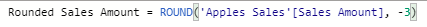For the second formula that is based on time, you will need:

• The field as a time
• What amount of time you want to round by

This formula doesn’t use the generic Round () function, but instead uses MRound (). This makes it possible to use a Time function to enter as the multiple.

The generic formula will look like this:

Round Time = MROUND (Field Name, Multiple)

A formula that has the information entered will look like this: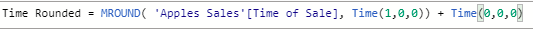We are using the second Time () function to make sure that the formula is seen as a time and not a numeric value.

Conclusion

Even though the round function shouldn’t be used in most situations, it helps to clear up a view of data when you only want to see the trend and are not looking into specifics. There are many other uses for the round function, and the best I have found is using it to simplify my time fields. You saw how this function can help readability, and I hope that this helps you understand how to better improve your report.